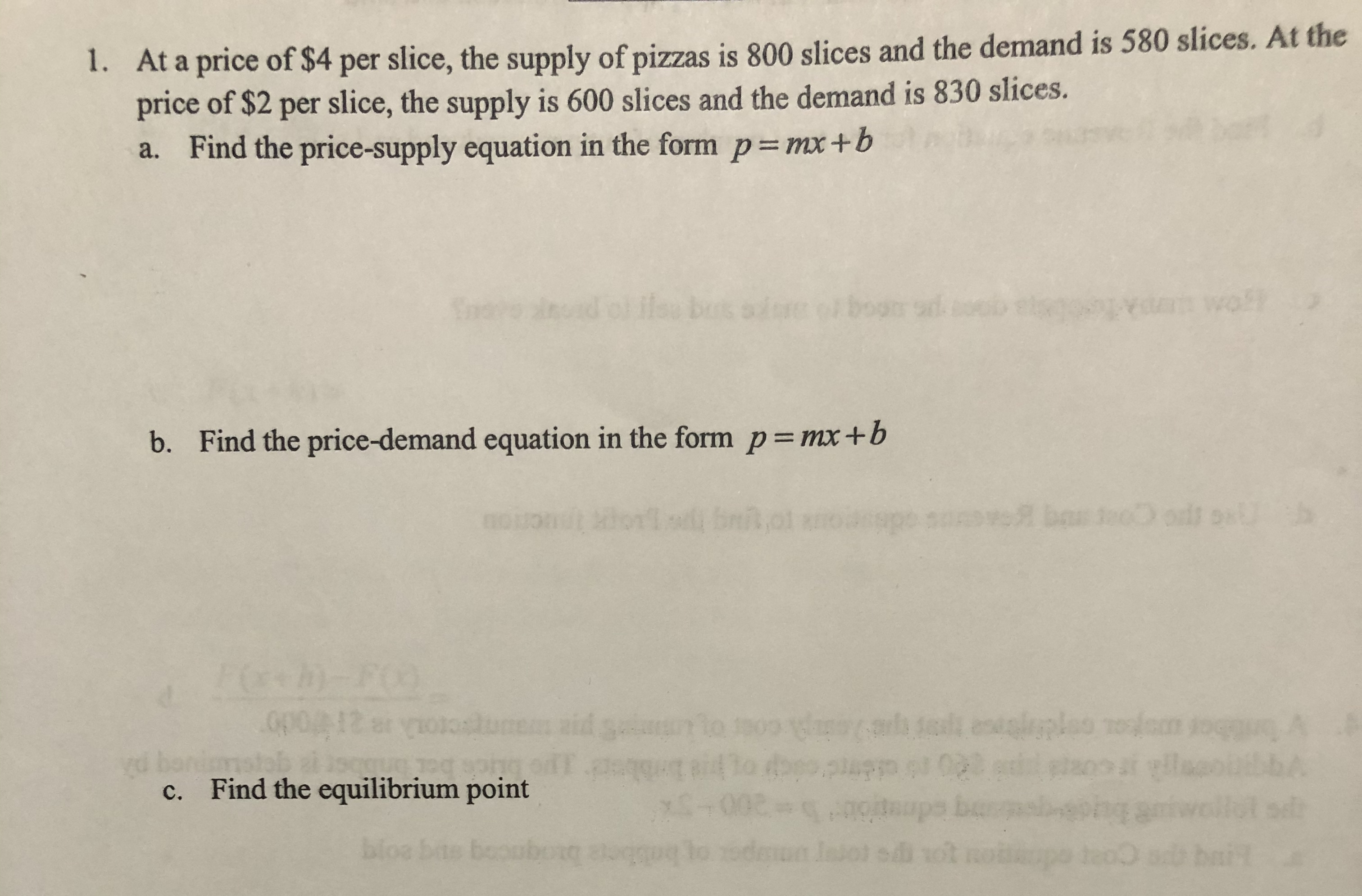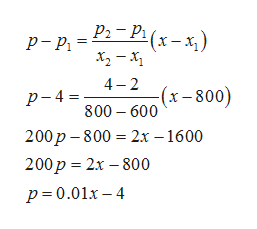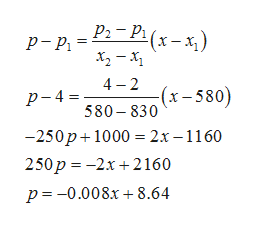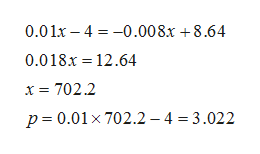# 1. At a price of \$4 per slice, the supply of pizzas is 800 slices and the demand is 580 slices. At theprice of \$2 per slice, the supply is 600 slices and the demand is 830 slices.a. Find the price-supply equation in the form p= mx+bilou sdreta woflbutsb. Find the price-demand equation in the form p= mx+bh)-FOopo12 eribior uoa onpdie t e coer o Spyd bonc. Find the equilibrium pointbloe bas bor

Question
96 viewshelp_outlineImage Transcriptionclose1. At a price of \$4 per slice, the supply of pizzas is 800 slices and the demand is 580 slices. At the price of \$2 per slice, the supply is 600 slices and the demand is 830 slices. a. Find the price-supply equation in the form p= mx+b ilou sdre ta wofl buts b. Find the price-demand equation in the form p= mx+b h)-FO opo12 er ibior uoa onpdie t e coer o Sp yd bon c. Find the equilibrium point bloe bas bor fullscreen
check_circle

Step 1

a) Since price-supply equation is linear , so we can use two-point form of equation by taking points (800,4) and (600,2)  to find the equation  as shown belowhelp_outlineImage TranscriptioncloseРа - Ра (х-х,) p- Pi = х, — х, 4- 2 (х-800) p- 4 = 800 – 600 200р - 800%- 2х —1600 200р %3D 2х — 800 р3D0.01х—4 fullscreen
Step 2

b) Since price-demand equation is linear , so we can use two-point form of equation by taking points (580,4) and (830,2)  to find the equation  as shown belowhelp_outlineImage TranscriptioncloseРа - Ра (х-х,) p- Pi х, — х, - 2 -(х - 580) 4 p- 4 = 580 - 830 —250р+1000 %3D 2х—1160 250р %3D —2х + 2160 р%3 -0.008х + 8.64 fullscreen
Step 3

c) Equilibrium point is when demand ...help_outlineImage Transcriptionclose0.01x – 4 = -0.008x +8.64 0.018x = 12.64 x = 702.2 p = 0.01x 702.2 – 4 = 3.022 fullscreen

### Want to see the full answer?

See Solution

#### Want to see this answer and more?

Solutions are written by subject experts who are available 24/7. Questions are typically answered within 1 hour.*

See Solution
*Response times may vary by subject and question.
Tagged in

### Equations and In-equations